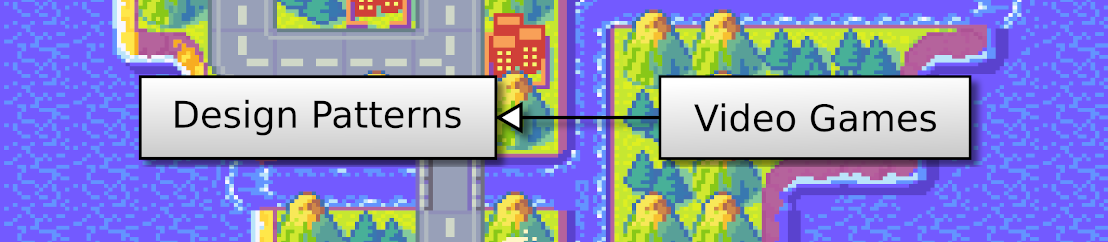## OpenGL 2D Facade (28): Point light

In this post, we start to work on lighting. In this first case, we want to create light around the main character.

This post is part of the OpenGL 2D Facade series

## Objective

We simulate the night through a change of colors on the whole map, except around the hero. That gives the illusion that the hero carries a light; it is not the best effect, we keep it simple for now:

## Simulate the night

### Alter pixel colors

We can alter the color of pixels using element-wise multiplication. In an OpenGL shader we can write it as:

``color *= colorFactors;``

which is the same as:

``````color.x *= colorFactors.x;
color.y *= colorFactors.y;
color.z *= colorFactors.z;
color.w *= colorFactors.w;``````

The x field in color contains the red channel, y the green channel, and z the blue channel. The weights in colorFactors are between 0 and 1 and lower values. For instance, if colorFactors is:

``````colorFactors.x = 0.8;
colorFactors.x = 0.6;
colorFactors.x = 0.4;
colorFactors.w = 1.0;``````

It lowers a bit the red channel (0.8), somewhat the green channel (0.6), and a lot the blue channel (0.4).

### Implementation

We add a new uniform variable `colorFactors` in the fragment shader, and we add the multiplication:

``````#version 330
in vec2 outputUV;
out vec4 color;
uniform sampler2D textureColors;
uniform vec4 colorFactors;         // New
void main() {
color = texture(textureColors, outputUV);
color *= colorFactors;       // New
}``````

We also add a new `colorsFactors` in the `LayerGroup` class with accessors. Then, the update of the new uniform variable is as usual:

``````colorFactorsShaderVar = glGetUniformLocation(self.__shaderProgramId, "colorFactors")
r, g, b = layerGroup.colorFactors

## Light around a point

We improve the fragment shader to apply the color change everywhere except around a point:

``````#version 330
in vec2 outputUV;
in vec4 worldVertex;
out vec4 color;
uniform sampler2D textureColors;
uniform vec4 colorFactors;
uniform vec2 pixelSize;
uniform vec3 lightProperties;
void main() {
// Color from tileset
color = texture(textureColors, outputUV);

// Compute distance to light
float worldLocationX = (worldVertex.x + 1) / pixelSize.x;
float worldLocationY = -(worldVertex.y - 1) / pixelSize.y;
float diffX = worldLocationX - lightProperties.x;
float diffY = worldLocationY - lightProperties.y;
float dist = sqrt(diffX * diffX + diffY * diffY);

// Apply color factors if far from light
color *= colorFactors;
}
} ``````

The new uniform variable `lightProperties` contains three values: (x, y) the pixel location of the light, and z the radius around this point.

Lines 14-18 compute the distance between the light location and the pixel currently processed by the shader. This location is in a new input variable `worldVertex`. It comes from the vertex shader that copies the vertex vector (see details after). The vertex location is in normalized coordinates (between -1 and 1), not in pixel coordinates. Consequently, lines 14-15 convert them using the "magic" formula we saw at the beginning of the series. We set the new uniform variable `pixelSize` with the `screenPixelWidth` and `screenPixelHeight` we compute during the creation of the window:

``````screenPixelWidth = 2 / float(screenWidth)
screenPixelHeight = 2 / float(screenHeight)``````

Lines 21-24 compare this distance to the radius of the light. We only apply the color change if we are far enough.

We update the vertex shader to get the vertex in the fragment shader:

``````#version 330
layout (location=0) in vec4 vertex;
layout (location=1) in vec2 inputUV;
out vec2 outputUV;
out vec4 worldVertex;    // New
uniform vec4 translation;
uniform vec2 uvShift;
void main() {
gl_Position = vertex + translation;
outputUV = inputUV + uvShift;
worldVertex = vertex;                   // New
} ``````